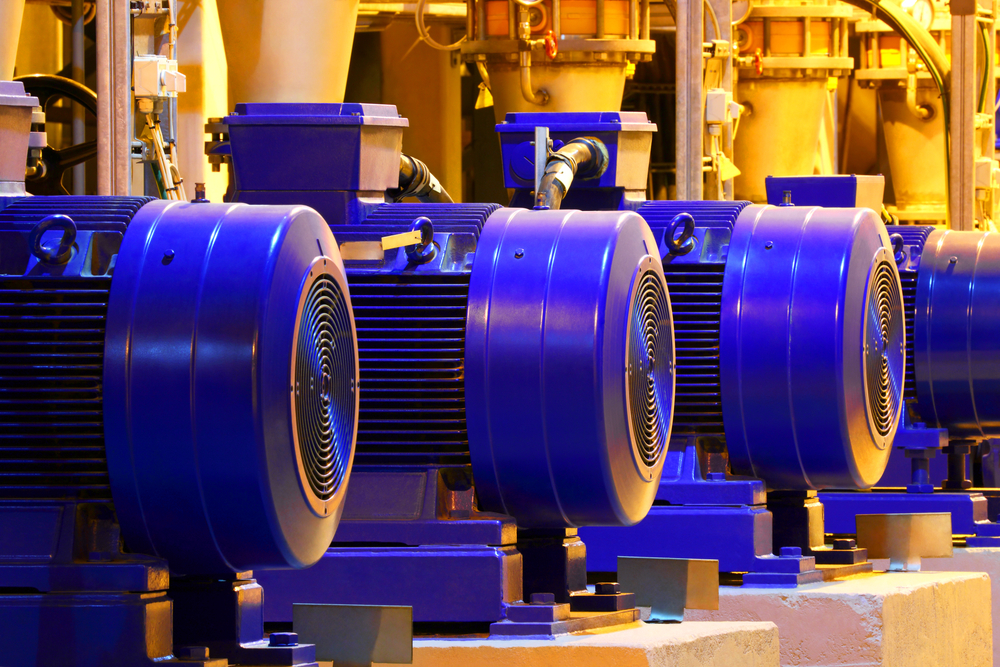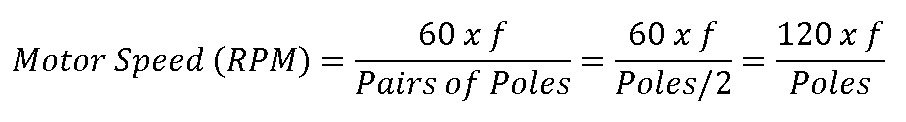# What Determines the Rotating Speed of a Motor?Author : Jahnavi Sajip
May 12, 2021• Home
• Blog
• What Determines the Rotating Speed of a Motor?
SHARE

Electric motors are characterized by their variety and wide range of sizes. There are fractional horsepower (hp) motors for small appliances, and motors with thousands of hp for heavy industrial use. Other specifications found in motor nameplates include their input voltage, rated current, energy efficiency and speed in RPM.

The rotating speed of an electric motor depends on two factors: its physical construction, and the frequency (Hz) of the voltage supply. Electrical engineers select the speed of a motor based on the needs of each application, similar to how the mechanical load determines the horsepower required.

## Make sure your building has the right electric motor for each application.## How the Voltage Frequency Relates to Motor Speed

Depending on the country, the power supply will have a frequency of 60 Hz or 50 Hz. Although a three-phase motor will rotate with both power inputs, there will be performance issues if a motor is specified for one frequency and used with the other.Since a 60Hz voltage supply switches polarity 20% faster than a 50Hz supply, a motor rated for 50Hz will spin at 20% higher rpm. Motor torque stays relatively constant, and a higher speed results in a higher shaft power. The motor also releases more heat, but the cooling fan also speeds up with the shaft, helping remove the extra heat. The motor also tends to draw more reactive current, which reduces its power factor.

Connecting a 60Hz motor to a 50Hz power supply is a more delicate matter. Reducing speed at the same voltage can saturate the magnetic core of the motor, increasing current and overheating the unit. The simplest way to prevent saturation is by lowering the input voltage, and ideally the V/Hz ratio should stay constant:

• A 60Hz motor operating at 50Hz is at 83.3% of its rated frequency.
• To keep the V/Hz ratio constant, the input voltage should also be reduced to 83.3%.
• If the electric motor normally operates at 240V and 60Hz, the input voltage at 50Hz should be 200V to keep a ratio of 4 V/Hz.

## Motor Wiring and Number of PolesA permanent magnet has two poles, but motors can be wired so their magnetic field has a higher number of poles. A two-pole motor completes a full revolution with one polarity change, while a four-pole motor only rotates 180° with one polarity switch. More poles result in a lower motor speed: if all other factors are equal, a 4-pole motor will rotate at half the speed of a 2-pole motor.

• A 60 Hz power supply changes polarity 60 times per second, and a two-pole motor will spin at 3,600 rpm when connected to this source. A four-pole motor will only rotate at 1,800 rpm.
• For 50 Hz motors, the speed is 3,000 rpm with 2 poles, and 1,500 rpm with 4 poles.

The concept can be summarized with the following equation:Using this equation, a 4-pole motor at 60Hz has a speed of 1,800 rpm, while a 6-pole motor at 50Hz has a speed of 1,000 rpm. However, this is actually the speed of the magnetic field, called the synchronous speed, which is not always equal to the shaft speed.

• In a synchronous motor, the rotor uses a permanent magnet or electromagnet to rotate at the calculated speed.
• On the other hand, an induction motor will operate slightly below the calculated rpm. This is how electromagnetic induction works, and should not be viewed as a malfunction.

If an electric motor has a nameplate speed of 1,800 rpm, one can conclude that the unit is a 4-pole synchronous motor rated for 60 Hz. On the other hand, if the nameplate speed has a lower value like 1,760 rpm, the unit is an induction motor.

A variable frequency drive can control motor speed by adjusting the input frequency, as its name implies. A VFD can also modulate voltage to keep the V/Hz ratio below the point where the magnetic core is saturated. Thanks to this feature, a VFD does not damage a motor even when speed is reduced below the nameplate value. The main drawback of VFDs is causing harmonic distortion, since they are nonlinear loads, but this can be compensated with harmonic filters.